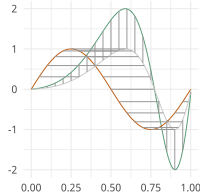# Elastic Functional Boxplots¶

Elastic Functional Boxplots

moduleauthor:: J. Derek Tucker <jdtuck@sandia.gov>

class boxplots.ampbox(fdawarp)[source]

This class provides amplitude boxplot for functional data using the SRVF framework

Usage: obj = ampbox(warp_data)

Parameters: warp_data (fdawarp) – fdawarp class with alignment data Q1 – First quartile Q3 – Second quartile Q1a – First quantile based on alpha Q3a – Second quantile based on alpha minn – minimum extreme function maxx – maximum extreme function outlier_index – indexes of outlier functions f_median – median function q_median – median srvf plt – surface plot mesh

Author : J. D. Tucker (JDT) <jdtuck AT sandia.gov> Date : 15-Mar-2018

construct_boxplot(alpha=0.05, k_a=1)[source]

This function constructs the amplitude boxplot using the elastic square-root slope (srsf) framework.

Parameters: alpha – quantile value (e.g.,=.05, i.e., 95%) k_a – scalar for outlier cutoff (e.g.,=1)
plot()[source]

plot box plot and surface plot

Usage: obj.plot()

class boxplots.phbox(fdawarp)[source]

This class provides phase boxplot for functional data using the SRVF framework

Usage: obj = phbox(warp_data)

Parameters: warp_data (fdawarp) – fdawarp class with alignment data Q1 – First quartile Q3 – Second quartile Q1a – First quantile based on alpha Q3a – Second quantile based on alpha minn – minimum extreme function maxx – maximum extreme function outlier_index – indexes of outlier functions median_x – median warping function psi_median – median srvf of warping function plt – surface plot mesh

Author : J. D. Tucker (JDT) <jdtuck AT sandia.gov> Date : 15-Mar-2018

construct_boxplot(alpha=0.05, k_a=1)[source]

This function constructs phase boxplot for functional data using the elastic square-root slope (srsf) framework.

Parameters: alpha – quantile value (e.g.,=.05, i.e., 95%) k_a – scalar for outlier cutoff (e.g.,=1)
plot()[source]

plot box plot and surface plot

Usage: obj.plot()